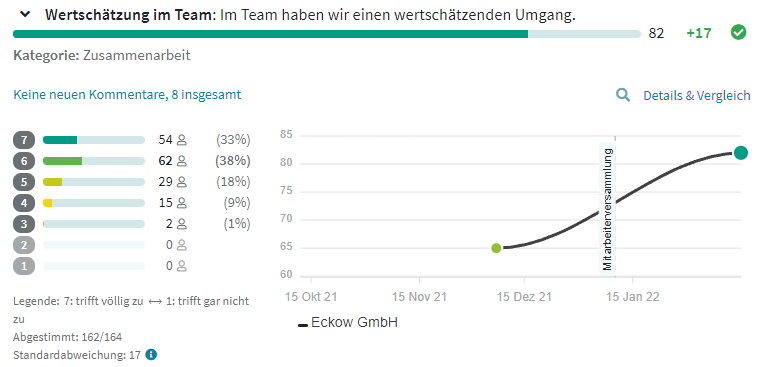Support-Center
Understanding the basics
1. Support-Center
2. Understanding the basics

# How does teamecho calculate the values?

## The teamecho Insights sentiment index is always composed of the most current values of all questions and categories. This way you always have a full overview of the mood in your teams.

teamecho Insights evaluates your quantitative results on three levels:

• Satisfaction index = consists of the average of the current values of all categories
• Category evaluation = consists of the mean value of the current values of all questions in this category
• Question evaluation = is calculated from the current answers to the question

### The calculations in detail

Are you wondering how a value is calculated and want to know exactly? Then join us for our little calculation talk:

teamecho Insights uses 7-point scales that are equidistantly distributed. That means each value on our scale is equidistant from adjacent values. As a team member, I can answer questions with values between 1 and 7, or give no answer.

After answering the scale values, a comment field for qualitative feedback always appears in teamecho surveys. However, here we explain the evaluation logic of the quantitative measurement values.

For the calculation of the displayed values, the scale points are converted to a scale from 0 to 100 - the answers to the teamecho Insights questions thus correspond from 0% to 100% agreement.

The distribution of agreement on a 7-point scale is accordingly:

• 1 = 0 % agreement
• 2 = 16,6 % agreement
• 3 = 33,3 % agreement
• 4 = 50 % agreement
• 5 = 66,6 % agreement
• 6 = 83,3 % agreement
• 7 = 100 % agreement

Now, to calculate the value of a question, we add up all the answers and the corresponding agreement values. Then we calculate the mean value of the answers and at the end we round to whole numbers. Here is a small calculation example:In the picture you can see the number of individual answers to the question "In the team we have an appreciative interaction". And now we calculate:

54*100 + 62*83,3 + 29*66,6 + 15*50 + 2*33,3 + 0*16,6 + 0*0

We multiply the number of responses by the corresponding approval value and add up all the responses.

The result = 13,312.6

In total, this question has 162 answers. Therefore we now calculate

13.312,6 / 162

The result = 82.2

Last but not least, a little rounding and we have the teamecho Score of this question: 82!

Looking for tips and tricks to analyze your results? You've come to the right place!